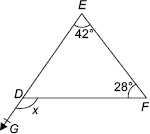# User ForumSubject :IMO    Class : Class 7

Find the measure of the angle x in the given figure.A 50°
B 70°
C 60°
D 30°

The answer is C and not B because :Angle EDF=180-42-28=120 Now , Sum of Angle GDF and EDF is 180. We know Angle EDF is 120 , so Angle GDF is 180-120=60 !